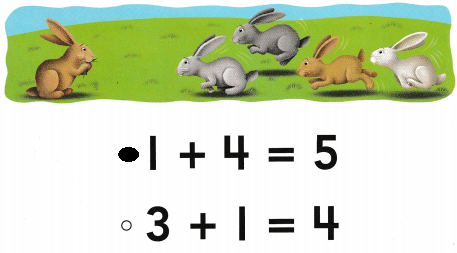# Texas Go Math Kindergarten Lesson 11.3 Answer Key Sums Up to 5

Refer to our Texas Go Math Kindergarten Answer Key Pdf to score good marks in the exams. Test yourself by practicing the problems from Texas Go Math Kindergarten Lesson 11.3 Answer Key Sums Up to 5.

## Texas Go Math Kindergarten Lesson 11.3 Answer Key Sums Up to 5

Explore

DIRECTIONS: Use counters to model the addition sentence. There are three birds on a branch. There is one bird flying. How many birds are there altogether? Trace and write the numbers and symbols to show how many birds in all.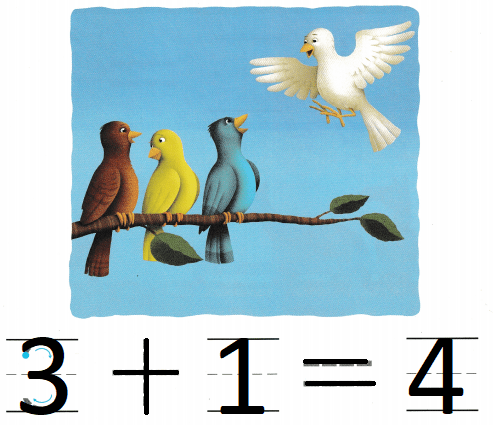Explanation:
There are three birds on a branch.
There is one bird flying.
3 + 1 = 4
so, there are 4 birds

Share and Show

DIRECTIONS: 1. There are two ducks swimming in a pond. Two more ducks join them. What is the total number of ducks? Write the number sentence. 2. There are two horses inside the fence. There is one horse outside the fence. How many horses are there? Write the number sentence.

Question 1.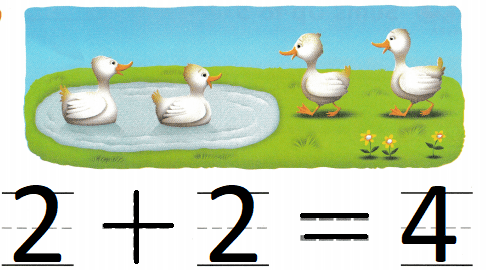Explanation:
There are two ducks swimming in a pond.
Two more ducks join them.
2 + 2 = 4
so, there are 4 ducks in all.

Question 2.Explanation:
There are two horses inside the fence.
There is one horse outside the fence.
2 + 1 = 3
so, there are 3 horses in all.

DIRECTIONS: 3. There are four fish swimming in the water. One more fish joins them. How many fish are there now? Write the number sentence. 4. There is one pig in the pigpen. There are two more pigs outside the pigpen. How many pigs are there? Write the number sentence.

Question 3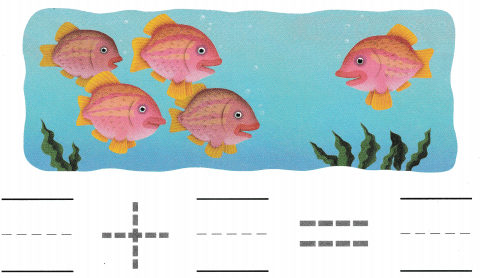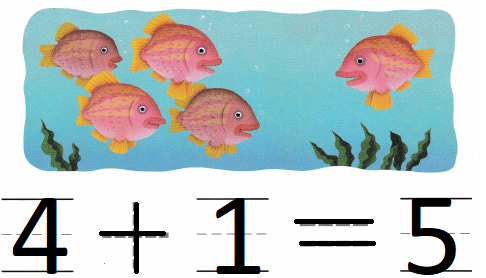Explanation:
There are four fish swimming in the water.
One more fish joins them.
4 + 1 = 5
so, there are 5 fishes in all.

Question 4.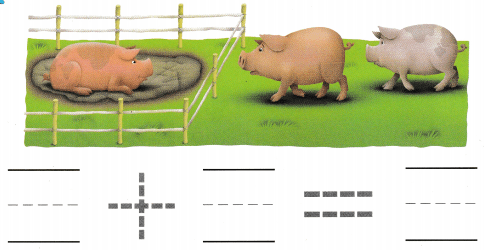Explanation:
here is one pig in the pigpen.
There are two more pigs outside the pigpen
1 + 2 = 3
so, there are 3 pig in pig pen

HOME ACTIVITY • Show your child a set of 1 to 5 objects such as pencils or markers. Have your child add one more to the set and count to tell how many in all.Explanation:
There are 5 markers with the kid 1 more is added to it
5 + 1 = 6
so, there are 6 markers in all.

DIRECTIONS: 5. Ani collects three rocks. Jenna collects two rocks. How many rocks do they collect together? Draw to show the rocks. Write to complete the addition sentence. 6. Choose the correct answer. Which number sentence shows what is happening in the picture?

Problem Solving

Question 5.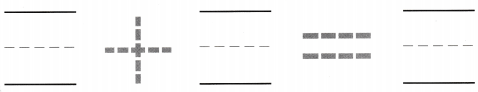Explanation:
Ani collects three rocks. Jenna collects two rocks.
2 + 3 = 5
so, they  collected 5 rocks  together

Question 6.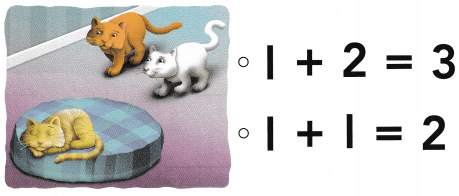Explanation:
One cat is sleeping and 2 more cats joined them
1 + 2 = 3
so, there are 3 cats in all.

### Texas Go Math Kindergarten Lesson 11.3 Homework and Practice Answer Key

DIRECTIONS: 1. There are three frogs sitting on a log. One more frog joins them. What is the total number of frogs? Write the number sentence. 2. There is one sheep eating grass. Four more sheep join. What is the total number of sheep? Write the number sentence.

Question 1.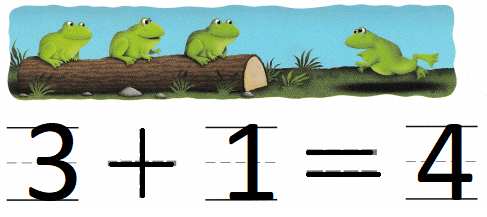Explanation:
There are three frogs sitting on a log.
One more frog joins them
3 + 1 = 4
so, there are 4 frogs.

Question 2.Explanation:
1 sheep eating the grass
and 4 sheep joined them
1 + 4 = 5
so, there are 5 sheep in all

DIRECTIONS: Choose the correct answer. 3-4. Which number sentence shows what is happening in the picture?

Lesson Check

Question 3.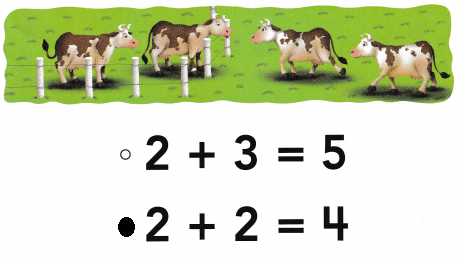Explanation:
2 cows are in pen
and 2 more joined them
2 + 2 = 4
so, there are 4 cows.

Question 4.